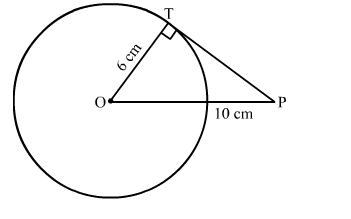# In the given figure, PT is a tangent to a circle with centre O. If OT = 6 cm and OP = 10 cm,`
Question:

In the given figure, PT is a tangent to a circle with centre O. If OT = 6 cm and OP = 10 cm, then the length of tangent PT is
(a) 8 cm
(b) 10 cm
(c) 12 cm
(d) 16 cmSolution:

In right triangle PTO
By using Pythagoras theorem, we have
PO2 = OT2 + TP2
⇒ 102 = 62 + TP2
100 = 36 + TP2
TP2 = 64
⇒ TP = 8 cm
Hence, the correct answer is option (a).